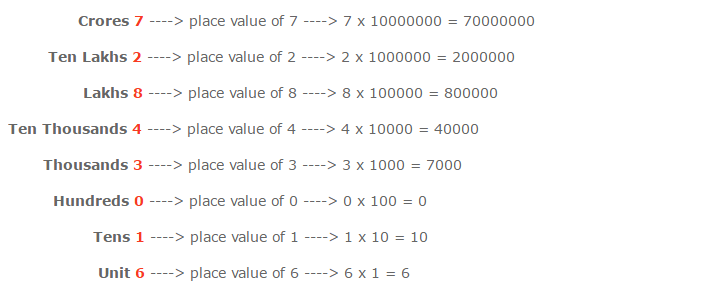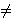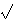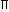Number System

A system in which we study different types of numbers, their relationship and rules govern in them is called as number system.

In the Hindu-Arabic system, we use the symbols 0, 1, 2, 3, 4, 5, 6, 7, 8 and 9. These symbols are called digits. Out of these ten digits, 0 is called an insignificant digit whereas the others are called significant digits.

Numerals

A mathematical symbol representing a number in a systematic manner is called a numeral represented by a set of digits.

Face Value and Place Value of the Digits in a Number

Face Value

In a numeral, the face value of a digit is the value of the digit itself irrespective of its place in the numeral.

For Example : In the numeral 486729, the face value of 8 is 8, the face value of 7 is 7, the face value of 6 is 6, the face value of 4 is 4, and so on.

Place Value (or Local Value)

In a numeral, the place value of a digit changes according to the change of its place.

Look at the following to get the idea of place value of digits in 72843016.It is clear from the above presentation that to obtain the place value of a digit in a numeral, we multiply the digit with the value of its place in the given numeral.

Types of Numbers

1. Natural Numbers

Natural numbers are counting numbers. They are denoted by N.

For Example          N = {1,2,3,...}.

* All natural numbers are positive.

* Zero is not a natural number. Therefore, 1 is the smallest natural number.

2. Whole Numbers

All natural numbers and zero form the set of whole numbers. Whole numbers are denoted by W.

For Example         W = {0,1,2,3,...}

* Zero is the smallest whole number.

* Whole numbers are also called as non-negative integers.

3. Integers

Whole numbers and negative numbers form the set of integers. They are denoted by I.

For Example    I = {...,-4,-3,-2,-1,0,1,2,3,4,...}

Integers are of two types.

(i). Positive Integers - Natural numbers are called as positive integers. They are denoted by  I+.

For Example     I+  = {1,2,3,4,...}

(ii). Negative Integers - Negative of natural numbers are called as negative integers. They are denoted by I-

For Example   I- = {-1,-2,-3,-4,...}

Note: '0' is neither +ve nor -ve integer.

4. Even Numbers

A counting number which is divisible by 2, is called an even number.

For Example    2, 4, 6, 8, 10, 12,...etc

* The unit's place of every even number will be 0, 2, 4, 6 or 8.

5. Odd Numbers

A counting number, which is not divisible by 2, is known as an odd number.

For Example   1, 3, 5, 7, 9, 11, 13, 15, 17, 19,...etc.

* The unit's place of every odd number will be 1, 3, 5, 7 or 9.

6. Prime Numbers

A counting numbers is called a prime number when it is exactly divisible by, 1 and itself.

For Example      2, 3, 5, 7, 11, 13, ...etc.

* 2 is the only even number which is prime.

* A prime number is always greater than 1.

* 1 is not a prime number. Therefore, the lowest odd prime number is 3.

* Every prime number greater than 3 can be represented by 6n + 1, where n is integer.

7. Composite Numbers

Composite numbers are non-prime natural numbers. They must have atleast one factor apart from 1 and itself.

For Example     4, 6, 8, 9, etc.

* Composite numbers can be both odd and even.

* 1 is neither a prime number nor composite number.

8. Co-primes

Two natural numbers are said to be co-primes, if their HCF is 1.

For Example      (7, 9),(15, 16)

* Co-prime numbers may or may not be prime.

9. Rational Numbers

A number that can be expressed as p/q is called a rational number, where p and q are integers and q0.

For Example  3/5, 7/9, 8/9, 13/15 etc.

10. Irrational Numbers

The numbers that cannot be expressed in the form of p/q are called irrational numbers, where p and q are integers and q0.

For Example2,3,7, etc.is an irrational number as 22/7 is not the actual value ofbut it is its nearest value.

* Non-periodic infinite decimal fractions are called as irrational number

11. Real Numbers

Real numbers include rational and irrational numbers both.

For Example    7/9,2,5,,  8/9 etc.

* Real numbers are denoted by R.

Important Formulas of Number System

Formulas of Number Series

1. 1 + 2 + 3 + 4 + 5 + … + n = n(n + 1)/2
2. (12 + 22 + 32 + ..... + n2) = n ( n + 1 ) (2n + 1) / 6
3. (13 + 23 + 33 + ..... + n3) = (n(n + 1)/ 2)2
4. Sum of first n odd numbers = n2
5. Sum of first n even numbers = n (n + 1)

Mathematical Formulas

1. (a + b)(a - b) = (a2 - b2)
2. (a + b)2 = (a2 + b2 + 2ab)
3. (a - b)2 = (a2 + b2 - 2ab)
4. (a + b + c)2 = a2 + b2 + c2 + 2(ab + bc + ca)
5. (a3 + b3) = (a + b)(a2 - ab + b2)
6. (a3 - b3) = (a - b)(a2 + ab + b2)
7. (a3 + b3 + c3 - 3abc) = (a + b + c)(a2 + b2 + c2 - ab - bc - ac)
8. When a + b + c = 0, then a3 + b3 + c3 = 3abc
9. (a + b)n = an + (nC1)an-1b + (nC2)an-2b2 + … + (nCn-1)abn-1 + bn

Shortcuts for number divisibility check

1. A number is divisible by 2, if its unit's digit is any of 0, 2, 4, 6, 8.
2. A number is divisible by 3, if the sum of its digits is divisible by 3.
3. A number is divisible by 4, if the number formed by the last two digits is divisible by 4.
4. A number is divisible by 5, if its unit's digit is either 0 or 5.
5. A number is divisible by 6, if it is divisible by both 2 and 3.
6. A number is divisible by 8, if the number formed by the last three digits of the given number is divisible by 8.
7. A number is divisible by 9, if the sum of its digits is divisible by 9.
8. A number is divisible by 10, if it ends with 0.
9. A number is divisible by 11, if the difference of the sum of its digits at odd places and the sum of its digits at even places, is either 0 or a number divisible by 11.
10. A number is divisible by 12, if it is divisible by both 4 and 3.
11. A number is divisible by 14, if it is divisible by 2 as well as 7.
12. Two numbers are said to be co-primes if their H.C.F. is 1. To find if a number, say y is divisible by x, find m and n such that m * n = x and m and n are co-prime numbers. If y is divisible by both m and n then it is divisible by x.

Shortcuts for 'recurring decimal to fraction' conversion

1. For recurring decimals of format '0.abababab...' (ab repeats), equivalent fraction will be "repeating group (here ab)"/"as many 9's as the number of digits in repeating group"
2. For recurring decimals of format '0.abbbbb...' (b repeats), equivalent fraction will be (entire decimal group - non-repeating decimal group)/(as many 9's as the number of repeating digits in the decimal part with as many 0's as the number of non-repeating digits in the decimal part)

TIPS on cracking Aptitude Questions on Number Systems

Tip #1: Factorize the expression

1.    (a + b)(a - b) = (a2 - b2)

2.    (a + b)2 = (a2 + b2 + 2ab)

3.    (a - b)2 = (a2 + b2 - 2ab)

4.    (a + b + c)2 = a2 + b2 + c2 + 2(ab + bc + ca)

5.    (a3 + b3) = (a + b)(a2 - ab + b2)

6.    (a3 - b3) = (a - b)(a2 + ab + b2)

7.    (a3 + b3 + c3 - 3abc) = (a + b + c)(a2 + b2 + c2 - ab - bc – ac)

Question: 297 x 297 =?

Solution:

297 x 297 = (300 – 3) (300 – 3) = (300 – 3)2 = 3002 + 32 – 2x3x300

=   297 x 297 = 90000 + 9 – 1800 = 88209

Question: 753 x 753 + 247 x 247 - 753 x 247 = ?

753 x 753 x 753 + 247 x 247 x 247

Solution:

Let 753 = a and 247 = b. Then the fraction can be written as:

a2 + b2 – ab =        a2 – ab + b2      =    1    =   1 .

a3 + b3         (a + b) (a2 – ab + b2)    a + b    1000

Tip #2: Use divisibility tests where applicable

Question: How many 3-digit numbers are completely divisible 6?

Solution:

Smallest 3-digit number divisible by 6:

100 is not divisible by 3.

101 is not divisible by 2.

102 is divisible by both 2 and 3 and hence, by 6.

Largest 3-digit number divisible by 6:

999 is not divisible by 2.

998 is not divisible by 3.

997 is not divisible by 2.

996 is divisible by both 2 and 3 and hence, divisible by 6.

Thus, the numbers form an AP with a = 102, d = 6, l = 996, n = ?

102 + (n – 1)6 = 996

=    6n = 996 – 102 + 6 = 900

=    n = 900 / 6 = 150

Question: If “481d673” is divisible by 9, what should be the smallest whole number in place of d?

Solution:

For the number to be divisible by 9, the sum of the digits should be divisible by 9.

4+8+1+6+7+3 = 29

The whole number just largest than 29 that is divisible by 9 is 36.

Thus, d = 36 – 29 = 7

Tip #3: Apply the rules of Binomial Theorem

n

(x + y)n = ? nCrx(n – r)yr

r=0

1.    (1 – x)-1 = 1 + x + x2 + x3 + …  where x is between 0 and 1

2.    (1 + x) -1 = 1 – x + x2 – x3 + …    where x is between 0 and 1

3.    xn + 1 is divisible by x + 1 for all odd values of n.

Proof: Let us prove this formula using the method of induction.

When n=1, xn + 1 = x+1 which is divisible by (x+1). When n=2, x2 + 1 is not divisible by (x+1).

Let x2k+1 + 1 be divisible by (x+1).

When n = 2k+3 (the next odd number after 2k + 1)

x2k+3 + 1 = x2(x2k+1 + 1) + (1 - x2) = x2(x2k+1 + 1)+(1+x)(1-x), which is divisible by (x+1).

Thus, xn + 1 is divisible by x + 1 when n is odd.

xn – 1 is divisible by x + 1 only when n is even. [Proof is similar to the one above]

Question: What will be remainder when (6767 + 67) is divided by 68?

Solution:

From Binomial Theorem, we know, xn + 1 is divisible by x + 1 only when n is odd.

6767 + 67 = (6767 + 1) + 66

Thus, remainder = 66

Question: Find the value of (0.999)3 correct to 3 decimal places.

Solution:

(0.999) 3 = (1 – 0.001) 3

= 13 - C(3,1)12(0.001) + C(3,2)1(0.001)2 - (0.001)3

= 1 - 0.003 = 0.997 (neglecting 3rd and 4th terms since they are « 0.001)

Tip #4: Remember the rules for division

Dividend = (Divisor x Quotient) + Remainder

Any recurring decimal can be written as the sum of a non-recurring decimal and a fraction of the recurring  portion of the decimal out of (10n – 1).

Ex: 0.125125125…= 0.125 = 125/999 and 7.2341341341… = 7.2 + 341/9999

Question: Express 0.232323..... As a rational number.

Solution:

0.232323… = 23/99.

Question: A student mistook the divisor as 12 instead of 21 and obtained 35 as quotient and reminder as 0. What is the correct quotient?

Solution:

Let the dividend be x.

Then, x / 12 = 35

=    x = 12 x 35

=    Correct quotient = (12 x 35 / 21) = 4 x 5 = 20.

Question: Find the smallest 6 digit number exactly divisible by 111.

Solution:

Smallest 6 digit number = 100000

When 100000 is divided by 111, the quotient is 900 and the remainder is 100.

The number would be divisible by 111 if the difference between 99900 (the number just lesser than 100000 that is divisible by 111) and the next divisible number is 111.

Thus, the smallest 6 digit number divisible by 111 is 99900 + 111 = 100011.

Tip #5: Memorize the formulae for sum of powers and apply where suitable

1.    1 + 2 + 3 + ….. + n = n (n + 1) / 2

2.    12 + 22 + 32 + ….. + n2 = n (n + 1)(2n + 1) / 6

3.    13 + 23 + 33 + …... + n3 = [ n (n + 1) / 2 ] 2

Question: (51 + 52 + 53 + ... + 100) =?

Solution:

(51 + 52 + 53 + ….. + 100) = (1 + 2 + 3 + ….. + 100) – (1 + 2 + 3 + ….. + 50)

= 100(100 + 1) - 50(50 + 1)

2                   2

= 5050 – 1275

= 3775.

Question: (22 + 42 + 62 + ... + 202) =?

Solution:

(22 + 42 + 62 + ... + 202) = 22(12 + 22 + 32 + ….. + 102)

= 22 x 10(10 + 1) (2•10 + 1)

6

= 4 x 10 x 11 x 21 / 6

= 1540.